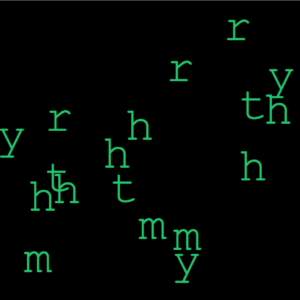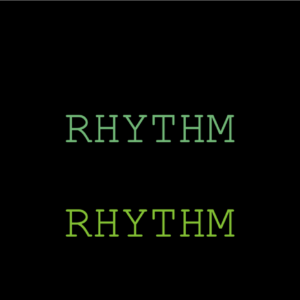Description:

Create a generative typography/text output.

Process:

In the beginning, I just wanted to play with letters and characters. I thought I would use noise to make a pattern as I did in my previous assignment, but randomness in this assignment suited better. So I started by writing a simple text message in the center of the screen, then I split the message into characters by using an array. Then I played around to make the characters randomly move around the screen, so it looked like the letter was flying in the air. This is what it looked like.Then, this looked boring, so I decided to add the key pressed function into it. So every time I would press the key pressed, my name would appear. The name would change its color and randomly disappear making it look nice and interactive. This is what it looked like.Overall, I would say I wanted to try some other things as well, but I did not know the process for some things, so in the end, I ended up experimenting and made this.

The final video looks like this:

This is the entire code:

```// Intro to IM - Assignment #4
// Rhythm Kukreja (rk3781@nyu.edu)

//setting the variables below
String letter = "rhythmrhythmrhythm";
String letter2 = "RHYTHM";
PFont font;
float x,y;
float hr, vr;
float w = 40;
float h = 40;
float r = 100;
char[] charArray;

void setup(){
frameRate(10);//reducing the framerate for the random effect in the beginning
size(640, 640);

charArray = new char[letter.length()];//to separate the words by character
for(int i=0; i<letter.length(); i++){
charArray[i] = letter.charAt(i);
}

background(255);
hr = textWidth(letter) / 2;
vr = (textAscent() + textDescent()) / 2;
font = createFont("Courier New", 100, true);
textFont(font);
textAlign(CENTER);

x = height/2;
y = width/2;
}

void draw(){
fill(0);
rect(0, 0, width, height);
float xpos=0; float ypos=0;

if (keyPressed){// the key is pressed we see the text that changes color and disappers
for (int i = 0; i < letter2.length(); i++) {
int flag = int(random(0,100));
if(flag%2==1){
fill(random(0,200), random(180,200), random(50,180));
} else {
fill(0);
}
text(letter2, width/2, ((i*100)+100));
}

} else {    //if key is not pressed, then we see characters flying around randomly
fill(random(0,200), random(180,200), random(50,180));
for(int i=0; i<letter.length(); i++){
xpos = random(10,width-10); ypos = random(10,height-10);
text(charArray[i], xpos, ypos);
}
}
}```

1.Aaron says: